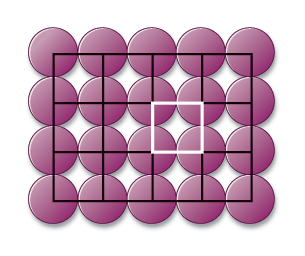# Problem: Calculate the packing efficiency of the two-dimensional lattice shown here:

🤓 Based on our data, we think this question is relevant for Professor Bloxton's class at TEMPLE.

###### FREE Expert Solution

Packing efficiency can be calculated as:

Notice that each atom is represented by a circle which has an area of πr2###### Problem Details

Calculate the packing efficiency of the two-dimensional lattice shown here: